The gauge foundations of special relativity*

Ó Danylchenko P.

SPE “GeoSystem”, Vinnitsa, Ukraine

Contact with author: pavlo@vingeo.com

It is shown here that Lorentz transformations are caused by gauge effect of motion on matter (principle nonobservability of effect of motion on matter). This gauge effect of motion is caused by interdependence and mutual determination of propagation velocity of interaction between matter elementary particles and of rate of course of matter proper (standard) time. The Lorentz transformations are derived without any linearity assumptions and being based only on the presence of relativistic shrinkage of the length of moving body and on clock desynchronization at its slowest transfer along this body.

## 1. Introduction

A lot of publications, being prejudiced fundamental postulates of Special Relativity (SR), have appeared recently. The most important among the brought-up problems is considering such substance as the physical vacuum (PV) to be a physical reality. After all, PV substitutes absolute ether of classical physics at rest in many ways. In addition, the possibility to work out the value of peculiar velocity of absolute motion of the Solar System by anisotropy of frequency of cosmic microwave background radiation contradicts with the established in the scientific literature opinion about the absence of special absolute frame of references of coordinates and time (FR), motionless relatively to the PV.

The aim of the present work is to show that seeming mutual incompatibility of the fundamental SR postulates with the presence of the undraggable by a moving body PV and corresponding to it the unique PVFR is caused only by imperfect understanding of physical essence of Lorentz transformations. The essence of these transformations (as it will be shown below) is in the precise mathematical mapping of gauge effect on matter and its space-time continuum (STC) [1, 2]. This gauge effect is the cause of principal nonobservability of any changes, which have realized in the objects and physical processes.

(As we know, influence of electric field on matter is realized only by spatial increments of electric potentials, not by the values of potentials. So we can gauge-transform these values. In analogy to this influence, influence of motion on matter, which we can observe in matter intrinsic FR as strengths of graviinertial (removable gravitational) field , is realized only by space-time increments of linear momentum, not by the values of linear momentum. Therefore, linear momentums of matter objects and, consequently, velocities (linear momentums are the functions of velocities) also can be gauge transformed by proceeding from observation of motion of this matter from one FR to the observation of it from another FR, as well as directly in the same FR - by their change in time, for example, for accelerating matter, which has rigid intrinsic FR (Möller FR) [3, 4].)

## 2. Derivation of the Lorentz transformations

As it was first shown by Fitzgerald and Lorentz , at the transformation of a state of body absolute rest into the state of steady inertial body motion relatively to the PV, uniquely definable shrinkage of the body size in the direction of its motion realizes itself in the absolute space. This shrinkage is connected with isobaric self-contraction of body matter . The self-contraction of matter is the result of adaptation of its molecules, atoms and elementary particles to changed conditions of their interactions.

Let the body moves at the absolute velocity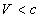relatively to the PV. Then longitudinal size Xij of the body, and consequently, the corresponding to it size of length standard, located on the body, shrink along the direction of motion in the same quantity of times: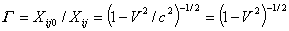.

Where: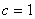considering the measuring of linear dimensions in light units of length. As a result of identical size shrinkage of measured objects, as well as of measurement instrumentation, motionless relatively to the moving body, no changes in geometry of its objects in body FR will be found out. And, consequently, transformations of linear and angular dimensions of the objects in the absolute space for the moving body and for the inertial FR (IFR), rigidly bound up with it, will be purely gauge. And the body will be gauge-self-deformed in this space. Due to such relativistic shrinkage of longitudinal sizes of the body the duration of absolute time of interaction between any two body points (or rather located there elementary particles):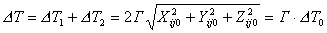,        (1)

will increase in G  times, where: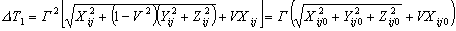,      (2)

and: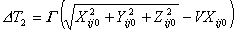,    (3)

- durations of time intervals of propagation of interaction waves accordingly in forward and reverse direction, and: Xij, Yij=Yij 0, Zij=Zij 0 - orthogonal projections of the segment between the interacting in the process of motion body points. As we see, the increase of duration of interaction time does not depend on the values of angles between the direction of body motion and directions of propagation of electromagnetic wave (virtual photon) in the forward and reverse move. And consequently, the repetition frequency of all the periodic physical processes, realizing in the moving body, including processes that used for chronometry, will decrease in G  times. And this means, that as the result of gauge effect of motion on matter, the time on the moving body (in the IFR, corresponding to it) will course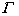times slower than on a body, motionless relatively to the PV. However, observers and instruments, motionless relatively to the body, will find no changes in realization of physical processes, which take place directly on the moving body.

Relativistic time dilation in IFR can’t be observed in principle by the intrinsic clock of IFR. Therefore, according to (2) and (3), time intervals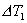and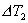must have the following durations by the clock of IFR: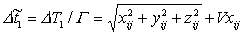,         (4)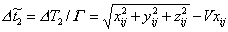,            (5)

where: xij≡Xij0, yij≡Yij0, zij≡Zij0 - projections dimensions of the moving body segments, observed in its IFR (in compliance with gauge transformations) with the same value as in case of their observation in state of body rest relatively to the PV. According to this, the value of the average velocity of propagation of interaction wave in the forward and reverse move will be observed in IFR the same as at its observation in the PVFR: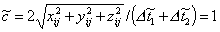,

This doesn’t allow us to find out mutual inequality of observed in the IFR and PVFR velocities of propagation of interaction wave or light, using location or interferometer.

Inequality of time intervals of the propagation of interaction wave in forward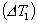and reverse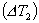move to its average value: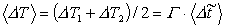,

is also impossible to be found out by IFR clock. After all, even in the case of slowest transfer of the clock along the shortest path from one point of the IFR to another, a mutual desynchronization of transferred and motionless in the IFR clocks realizes: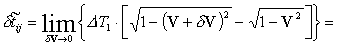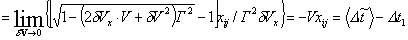,              (6)

where: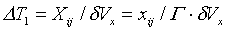,

and: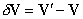is Galilei difference of vectors of absolute velocities of slowly transferred (V′) and motionless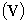in IFR clocks. This desynchronization is observed only in PVFR. And it compensates in the IFR the difference of the intrinsic time intervals Δt̃1 and Δt̃2, which proportionally synchronized with ΔT1 and ΔT2 correspondingly: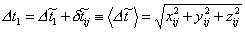,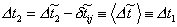.

As a result of this, a question appears, if equality in all the IFR points of proper quantum time (which determines their “age”) really does exist according to the observations from the PVFR. After all, in the process of increase of the value of velocity (till the value of uniform velocity) the motion of different points of the body realizes at unequal velocities . And this leads to the fact that “age” of different points of the body (measured by their quantum proper clock) will be unequal, according to (1). And consequently, the difference of points “age” will essentially depend on the law of motion of the body points during the process of reaching by them the equal values of absolute velocity. And as a result, standard time, determining the body points “age”, should be considered as their path-like proper (standard) time. To realize the possibility of analysis of dynamics of objects, which move in the IFR, coordinate-like intrinsic time (unified in all the points) [2, 4] must be introduced into it.

All of this is a sufficient reason for adoption of the conception of non-simultaneity of observation in the IFR of events, which realize simultaneously in the PVFR. The impossibility to observe in the IFR the desynchronization of the clock at its slowest transfer from one point of IFR to another: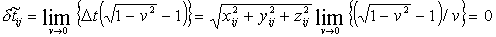shows up non-triviality of gauge transformation of time intervals. Time interval between the events, fixed in different points of the IFR by its intrinsic clock (which counts IFR coordinate time), is determined in PVFR, according to (6), by the following transformation: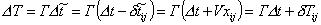,   (7)

where: δTij=Γ·V·xij - observed in the PVFR mutual desynchronization of events, which have simultaneously happened in the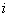and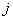points of the moving body IFR. Considering gauge transformation of size of the parallel to the direction of motion projection of segment xij, transformations of distance projections between these points at noncoinciding time moments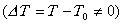, will be the following: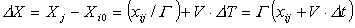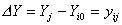,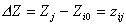,         (8)

According to (7) and (8) projections of velocity of the moving object at the transition from the IFR into PVFR and inversely will be transformed according to Lorentz rules . In that way, the velocity of light in free space will not depend in the IFR on absolute velocity of body, possessing this IFR. This, of cause, is connected with the equality of the velocity of light in free space to the velocity of propagation of the wave of electromagnetic interaction, which determines the frequency of this interaction between elementary particles of matter, and thus, the course rate of the IFR intrinsic time.

Consequently, Lorentz transformations are based on real shrinkage in absolute space of dimensions of objects along the direction of their motion, as well as on IFR intrinsic time dilation and desynchronization of slow-transferred clock, which are observed in the PVFR. Due to this, Lorentz transformations guarantee the impossibility to find out in the IFR any changes, which have happened to objects and physical processes, realizing in IFR, after the body has changed its state from absolute rest to its uniform motion relatively to the PV. In that way, the correctness of the first Einstein postulate about the sameness of realizing of all the physical phenomena in all the inertial systems is confirmed.

## 3. Effects, caused by Lorentz transformations

As the result of time dilation in the IFR the increase of the value of its effective velocity relatively to the PV (which is determined, according to (8), by a moving clock when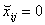) takes place: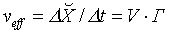.       (9)

Therefore, because of bigger intimes dash repetition frequency of motionless relatively to the PV linear scale, the value of its division will seem to betimes smaller in the IFR. And consequently, according to (7), the path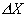, covered by the IFR in absolute space, which is observed in it as “contracted”, will be perceived in the IFR astimes smaller: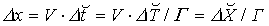.                                 (10)

On the other hand, according to (7) and (8), at the same IFR intrinsic time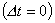its different points will be opposed to the PV points at the moments of absolute time, mutually detached by the interval: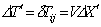(11)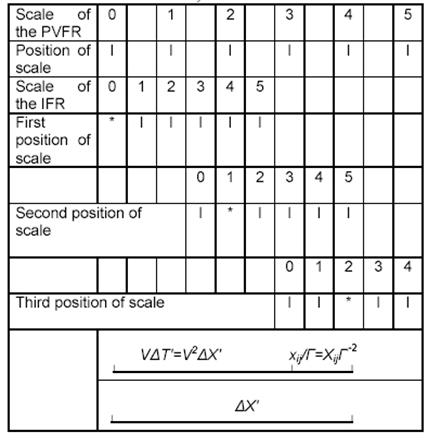These moments correspond (as it is shown in the figure, when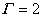) to different positions of the IFR relatively to the PV (events, simultaneous in the IFR, marked in the figure by the symbol “*”).

This will lead to observation in the IFR of “imaginary” shrinkage in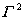times of dimensions of objects, motionless relatively to the PV. However, considering real shrinkage in absolute space of the dimensions of IFR objects intimes, the resulting shrinkage, observed in the IFR, of dimensions of objects, motionless relatively to the PV, will be only intimes: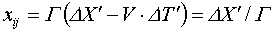.            (12)

That is why, the presence of real shrinkage in absolute space of dimensions of the IFR objects and the presence of “imaginary” shrinkage in the IFR space-time continuum of dimensions of objects, motionless relatively to the PV, leads to observation of dimensions of objects, motionless relatively to the IFR, in the PVFR and dimensions of objects, motionless relatively to the PV, in the IFR, as reduced in the same number of times. As the result of clock desynhronization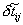at its slowest transfer into the IFR, “imaginary” absolute time dilation in Γ2 times in the IFR STC will also take place. However, because of the presence of real IFR intrinsic time dilation intimes in comparison with absolute time, the resulting absolute time dilation, observed in the IFR, will be only intimes: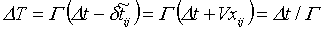,       (13)

where at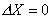: xij= – VΔt. Consequently, the presence of real time dilation in the IFR and “imaginary” dilation of absolute time leads to mutually observed time dilation on objects, moving in any of the FR. So, mutually observed identical shrinkages of objects and time dilations in mutually opposed FR are caused only by principle lack of mutually coincidence of time moments of reading in them of one of the two counts of space coordinates and principle lack of superposition of points of reading in them of one of the two counts of coordinate-like time accordingly. Incomprehension and neglect of this (together with indiscrimination of FR coordinate-like intrinsic time and path-like proper time of objects ) is the cause of origination in the SR of various imaginary paradoxes. And moreover, it causes false treatment of SR by some physicists as purely mathematic theory, which allows explaining observed physical phenomena only with some degree of conventionality.

## 4. Conclusions

In that way, Lorentz transformations correspond to gauge self -deformation in the absolute space of STC of uniformly moving body. And at this they image the impossibility of detection of any changes, which have realized in the objects and physical processes after replacement of state of absolute rest of the body to the state of its uniform motion relatively to the PV. And consequently, they image the principle impossibility of detection, in which of the two states the body is, using direct methods. However, the equality of any IFR with the PVFR, caused by this, by no means does not deny the natural occurrence of the unique PVFR, as well as of substance, motionless in it, - the PV (the absolute ether of classical physics), in which motion of objects, possessing mass, and propagation of electromagnetic waves take place. The PVFR in the Lorentz and Poincare groups of transformations is the element of not only set of the IFR, but also of sets of any other FR types of gauge-deformed and gauge-self-deformated bodies . Moreover PVFR is the unique common element of all the possible FR sets.

Gauge invariance of eigenvalue of the velocity of light (uniquely determined by matter proper quantum clock) in any of the groups of transformation is caused by interdependence and mutual determination of time course rate and of the velocity of propagation of interaction (equal to the velocity of light). In this way the interaction propagation velocity in space is set in the time. And the course rate of matter proper time, in its turn, depends on the velocity of propagation of interaction. After all, the rates of realization of any physical processes, used for chronometry, are proportional to velocity of propagation of interaction. That’s why it is impossible here to detect, which of the two physical parameters (time or the velocity of propagation of interaction) is initial (first-born). In that way, the impossibility of observing by the proper clock not only the change of course rate of time, measured by them, but also the change of velocity of propagation of interaction in the point of localization of the clock, is a property (postulated by Einstein only for IFR) of any other possible FR. And the relativity principle of SR is only the consequence of more fundamental principle – principle of gauge deformation of matter and its STC under the effect of motion and gravity .

### Reference list

.   Danylchenko P., Gauge Justification of Special Relativity, in: The Gauge-evolutional theory of the Creation, Ukraine, Vinnytsia, 1994, 1, 10

.   Danylchenko P, Foundations of the Gauge-evolutional theory of the Creation (space,time, gravitation and the Universe expansion), Ukraine,Vinnytsia, 1994

.   Danylchenko P., Nature of relativistic length shrinkage, in: Gauge-Evolutional Interpretation of Special and General Relativities, Vinnitsa, O. Vlasuk, 2004, p.3

.   Möller C., The Theory of Relativity, Oxford: Clarendon Press Oxford, 1972

.   Lorentz H., The theory of electrons, Leipzig: Teubner, 1916

.   Danylchenko P., Physical essence of twins paradox, in: Gauge-Evolutional Interpretation of Special and General Relativities, Vinnitsa, O. Vlasuk, 2004, p.25